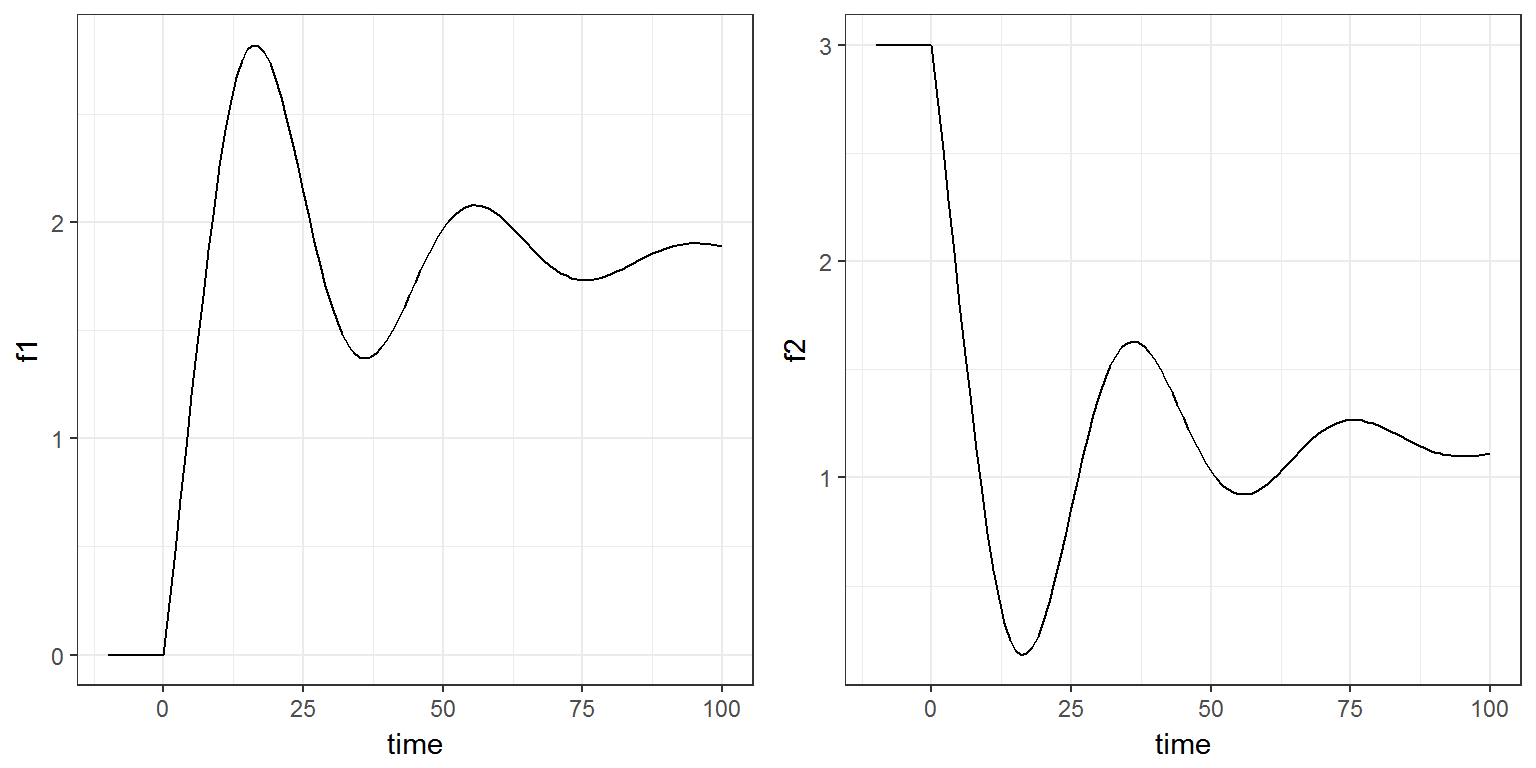R script: dde.R

$$\newcommand{\esp}{\mathbb{E}\left(#1\right)} \newcommand{\var}{\mbox{Var}\left(#1\right)} \newcommand{\deriv}{\dot{#1}(t)} \newcommand{\prob}{ \mathbb{P}\!(#1)} \newcommand{\eqdef}{\mathop{=}\limits^{\mathrm{def}}} \newcommand{\by}{\boldsymbol{y}} \newcommand{\bc}{\boldsymbol{c}} \newcommand{\bpsi}{\boldsymbol{\psi}} \def\pmacro{\texttt{p}} \def\like{{\cal L}} \def\llike{{\cal LL}} \def\logit{{\rm logit}} \def\probit{{\rm probit}} \def\one{{\rm 1\!I}} \def\iid{\mathop{\sim}_{\rm i.i.d.}} \def\simh0{\mathop{\sim}_{H_0}} \def\df{\texttt{df}} \def\res{e} \def\xomega{x} \newcommand{\argmin}{{\rm arg}\min_{#1}} \newcommand{\argmax}{{\rm arg}\max_{#1}} \newcommand{\Rset}{\mbox{\mathbb{R}}} \def\param{\theta} \def\setparam{\Theta} \def\xnew{x_{\rm new}} \def\fnew{f_{\rm new}} \def\ynew{y_{\rm new}} \def\nnew{n_{\rm new}} \def\enew{e_{\rm new}} \def\Xnew{X_{\rm new}} \def\hfnew{\widehat{\fnew}} \def\degree{m} \def\nbeta{d} \newcommand{\limite}{\mathop{\longrightarrow}\limits_{#1}} \def\ka{k{a}} \def\ska{k{\scriptscriptstyle a}} \def\kel{k{e}} \def\skel{k{\scriptscriptstyle e}} \def\cl{C{\small l}} \def\Tlag{T\hspace{-0.1em}{lag}} \def\sTlag{T\hspace{-0.07em}{\scriptscriptstyle lag}} \def\Tk{T\hspace{-0.1em}{k0}} \def\sTk{T\hspace{-0.07em}{\scriptscriptstyle k0}} \def\thalf{t{1/2}} \newcommand{\Dphi}{\partial_\pphi #1} \def\asigma{a} \def\pphi{\psi} \newcommand{\stheta}{{\theta^\star}} \newcommand{\htheta}{{\widehat{\theta}}}$$

# 1 Introduction

A system of delay differential equations (DDEs) can be implemented in a block EQUATION of the section [LONGITUDINAL] of a script Mlxtran.

Mlxtran provides the command delay(x,T) where x is a one-dimensional component and T is the explicit delay. Therefore, DDEs with a nonconstant past of the form \begin{align} \deriv{x} &= f(x(t),x(t-T_1), x(t-T_2), \ldots), \quad \text{for } \ \ t \geq 0\\ x(t) &= x_0(t) \quad \text{for } \ \ \min(T_k) \leq t \leq 0 \end{align} can be solved.

# 2 Example

We consider in this example a model where two functions $$f_1$$ and $$f_2$$ depending on a vector of parameters $$\phi=(a, b, \tau_1, \tau_2)$$ are defined by a system of DDEs. Here, $$\tau_1$$ and $$\tau_2$$ are delays.

\begin{align} \deriv{f_1} &= a\, f_2(t-\tau_2) - b\, f_1(t-\tau_1) \\ \deriv{f_2} &= b\, f_1(t-\tau_1) - a\, f_2(t-\tau_2) \end{align}

with the following initial condition: \begin{align} f_1(t) &= \max(10 + t, 0) \quad {\rm for } \ t\leq 0 \\ f_2(t) &= 3 \ \ \quad {\rm for } \ t\leq 0 \end{align}

This model can easily be implemented with Mlxtran:

dde.model <- inlineModel("
[LONGITUDINAL]
input = {a, b, tau1, tau2}

EQUATION:
t0 = 0
f1_0 = 10+t
f2_0 = 3
ddt_f1 = a*delay(f2,tau2) - b*delay(f1,tau1)
ddt_f2 = b*delay(f1,tau1) - a*delay(f2,tau2)
")

We will use Simulx for evaluating $$f_1$$ and $$f_2$$ every hour between time -10h and time 100h, with $$a=0.08$$, $$b=0.05$$, $$\tau_1=5$$ and $$\tau_2=10$$:

p <- c(a = 0.08, b  = 0.05, tau1 = 5, tau2 = 10)

out <- list(name=c('f1', 'f2'), time=seq(-10,100,by=1))

res <- simulx(model     = dde.model,
parameter = p,
output    = out)

plot1=ggplot(data=res$f1, aes(x=time, y=f1)) + geom_line() plot2=ggplot(data=res$f2, aes(x=time, y=f2)) + geom_line()
gridExtra::grid.arrange(plot1, plot2, ncol=2)